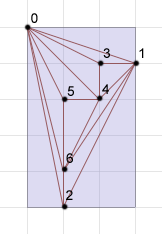### Problem Statement

We all know that a planar graph is one that you can draw without having any edges crossing. Perhaps less well known is that a planar graph can always be drawn with vertices on lattice points and with edges as straight lines between connected vertices.

You will be given a String[], graph, representing a planar undirected graph, where the jth character of element i of graph indicates whether vertices i and j are connected ('T' for true, 'F' for false). Your task is to find the area of the smallest rectangular box that the vertices can be placed in so that they are located on lattice points with non-overlapping straight edges between connected vertices.

### Definition

 Class: DrawPlanar Method: minArea Parameters: String[] Returns: int Method signature: int minArea(String[] graph) (be sure your method is public)

### Notes

-Lattice points are ones with integer coordinates.

### Constraints

-graph will contain between 1 and 7 elements, inclusive.
-Each element of graph will contain the same number of characters as there are elements in graph.
-Each character in graph will be 'T' or 'F'.
-Character i in element i of graph will be 'F'.
-Character i in element j of graph will be equal to character j in element i.
-The graph will be planar.

### Examples

0)

 `{"F"}`
`Returns: 0`
 With just one vertex, a single point suffices to draw the graph, so the area is 0.
1)

 ```{"FTF", "TFF", "FFF"}```
`Returns: 0`
 In this case, we can draw the vertices all in a line, again with area 0.
2)

 ```{"FTT", "TFT", "TTF"}```
`Returns: 1`
 Here, we can select any three corners of a 1x1 square.
3)

 ```{"FTTT", "TFTT", "TTFT", "TTTF"}```
`Returns: 4`
4)

 ```{"FTTTTTT", "TFTTTFT", "TTFFFFT", "TTFFTFF", "TTFTFTT", "TFFFTFT", "TTTFTTF"}```
`Returns: 15`#### Problem url:

http://www.topcoder.com/stat?c=problem_statement&pm=4846

#### Problem stats url:

http://www.topcoder.com/tc?module=ProblemDetail&rd=8077&pm=4846

lars2520

#### Testers:

PabloGilberto , Yarin , vorthys , Olexiy

#### Problem categories:

Brute Force, Graph Theory, Recursion